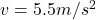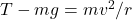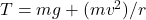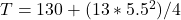## A heavy ball with a weight of 130 N is hung from the ceiling of a lecture hall on a 4.0-{\rm m}-long rope. The ball is pulled to one side an

Question

A heavy ball with a weight of 130 N is hung from the ceiling of a lecture hall on a 4.0-{\rm m}-long rope. The ball is pulled to one side and released to swing as a pendulum, reaching a speed of 5.5 m/s as it passes through the lowest point. What is the tension in the rope at that point?

in progress 0
1 month 2021-08-15T21:03:08+00:00 2 Answers 0 views 0

T = 228.3N

Explanation:

We are given:

w = 130Nr = 4.0m

We take g = 10

Therefore:

w = m×g

130 = m × 10

m = 130/10

m = 10

At the lowest point,we use the equation:;

Since we are asked to find tension T, we now have:Therefore, substituting figures in the equation, we have:;

T = 228.3N

The tension in the rope is 228.3N

Explanation: The weight of the ball will be equal to the product of its mass and acceleration due to gravity.

weight, w = mg

Then we can get the mass.

130 = mX10

m = 13 kg

Now let’s consider all the forces at the lowest point.

The sum of the forces will be equal to centripetal force because it swings.

Therefore:

T – mg = mv^2 / r

T = 130 + (13 X 5.5^2)/4.0 = 130 + 98.3 = 228.3 N